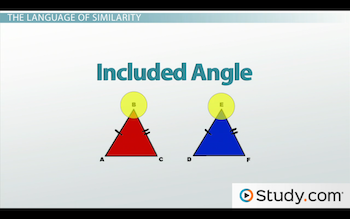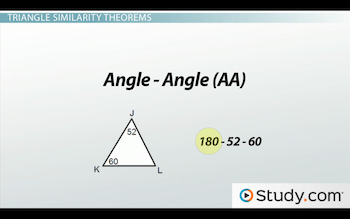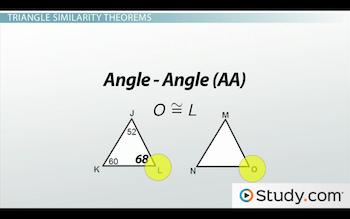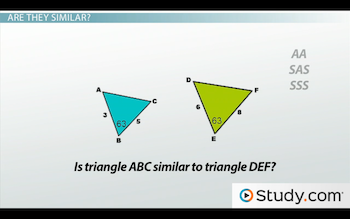# How to Identify Similar Triangles

An error occurred trying to load this video.

Try refreshing the page, or contact customer support.

Coming up next: Applications of Similar Triangles

### You're on a roll. Keep up the good work!

Replay
Your next lesson will play in 10 seconds
• 0:05 The Language of Similarity
• 1:35 Triangle Similarity Theorems
• 3:57 Are They Similar?
• 6:46 Lesson Summary

Want to watch this again later?

Timeline
Autoplay
Autoplay
Speed

#### Recommended Lessons and Courses for You

Lesson Transcript
Instructor: DaQuita Hester

DaQuita has taught high school mathematics for six years and has a master's degree in secondary mathematics education.

Similar triangles have the same characteristics as similar figures but can be identified much more easily. Learn the shortcuts for identifying similar triangles here and test your ability with a quiz.

## The Language of Similarity

Similar figures are figures that have the same shape but are different sizes. They have congruent corresponding angles and proportional corresponding sides. Similar triangles are a type of similar figure, and determining their similarity is much easier thanks to the triangle similarity theorems. These theorems, which are Angle - Angle (AA), Side - Angle - Side (SAS) and Side - Side - Side (SSS), make it possible to determine triangle similarity with minimal calculations. Before we go any further, let's review key terms that will help these theorems make sense.

When parts of a figure are corresponding, this means that they are in the same location in each figure. Sides are proportional when the ratios between the corresponding sides are congruent. So, if you create ratios or fractions comparing all of the corresponding sides, each will have the same value and reduce to the same number.

When discussing congruent or similar figures, the included angle is the angle formed by the congruent or proportional sides. In the triangle below, since side AB is congruent to side DE and side BC is congruent to side EF, then angles B and E are the included angles.## Triangle Similarity Theorems

Okay. Now that we're refreshed on vocabulary, let's further examine each of the similarity theorems.

We'll begin with Angle - Angle (AA). For two triangles to be similar by Angle - Angle (AA), two angles of one triangle are congruent to two angles of another triangle. Look at triangle JKL below. Angle J is 52 degrees and angle K is 60 degrees. If we subtract 52 and 60 from 180 (the total number of degrees that all angles in a triangle must add up to), we will see that angle L is 68 degrees.Now, look at triangle MNO below. If angle M is congruent to angle J, and angle N is congruent to angle K, what can we say about angles L and O? We can say that they are congruent as well. With three pairs of congruent angles, the triangles are the same shape but different sizes, meaning that their sides are proportional and the triangles are similar.For Side - Angle - Side (SAS), an angle of one triangle must be congruent to the corresponding angle of another triangle, and the lengths of the sides including these angles are in proportion. With congruent included angles, the proportional sides can't fluctuate, and the third side in both triangles must be a specific length. So, with two sides already proportional, the lengths of the third sides must also be proportional, proving triangle similarity.

The last theorem is Side - Side - Side (SSS), which means that the three sets of corresponding sides of two triangles are in proportion. If the ratios of all corresponding sides are equal, then the sides are similar and so are the triangles.

When determining which theorem proves similarity, don't overthink it; just look at the letters in each theorem. For triangles to be similar by Angle - Angle (AA), the measures of two angles in each triangle will be provided. If similar by Side - Angle - Side (SAS), then you will have the measures of two sides and the included angles of both triangles. For Side - Side - Side (SSS), you will have all three side lengths for both triangles. Let's practice.

## Are They Similar?

Is triangle ABC similar to triangle DEF?To unlock this lesson you must be a Study.com Member.

### Register to view this lesson

Are you a student or a teacher?

### Unlock Your Education

#### See for yourself why 30 million people use Study.com

##### Become a Study.com member and start learning now.
Back
What teachers are saying about Study.com

### Earning College Credit

Did you know… We have over 160 college courses that prepare you to earn credit by exam that is accepted by over 1,500 colleges and universities. You can test out of the first two years of college and save thousands off your degree. Anyone can earn credit-by-exam regardless of age or education level.